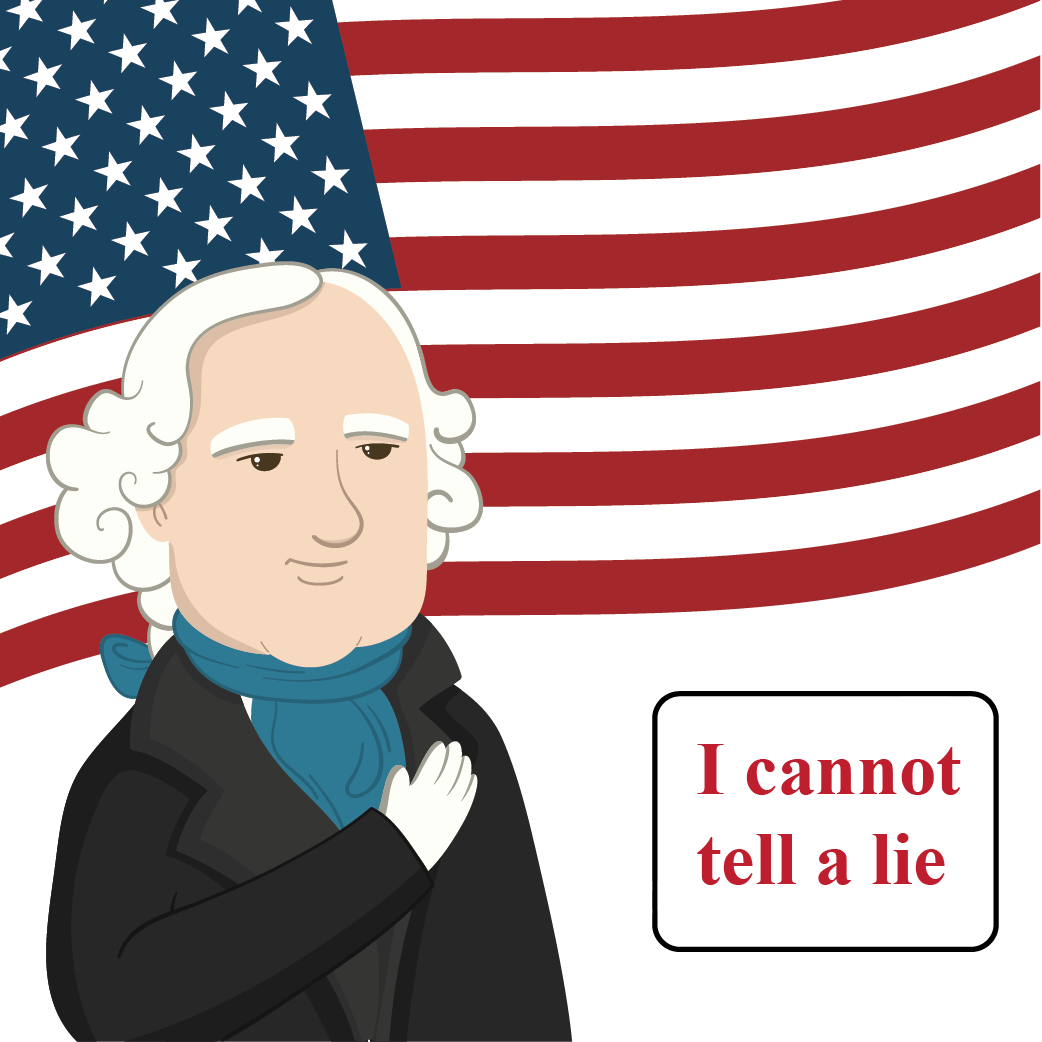# Tautology

Tautology

If I say, "I will give you $5 or I will not give you$5". What will you think about this comment?
I will always be true regardless of the fact that I give you some money or not.

The famous line by George Washington "I cannot tell a lie" is also another example of such statements.Such statements are well-explained and come under tautology.

So, let's get started to learn more about tautology examples, how to define tautology in math, and the tautology truth table.

## Lesson Plan

 1 What Is Tautology in Math? 2 Important Notes on Tautology 3 Thinking Out of the Box! 4 Solved Examples on Tautology 5 Interactive Questions on Tautology

## What Is Tautology in Math?

Definition

A logical combination of sentences that is always true, regardless of the truth or falsity of the constituent sentences, is known as a "tautology."

— Rudy Rucker

A tautology is a compound statement that is always true, no matter if the individual statements are false or true.

The word tautology is derived from a Greek word where ‘tauto’ stands for ‘same’ and ‘logy’ stands for ‘logic’.

In order to know if a given statement is a tautology, we need to construct a truth table and look at the truth value in the final column.

If all the values are true then it is a tautology.

A few simple examples of tautology are:

• Either you will jump or you will not jump.
• Henry is slim or Henry is not slim.
• Emanuel will dance or Emanuel will not dance.

All these statements are true, no matter what.

## Logic Symbols in Math

As mathematics is a logical subject, it uses logical statements to determine the answers.

To know whether a given statement is tautological or not, tautology logic needs to be true.

In Tautology, different logical symbols are used to present compound statements.

Here are the logic symbols, that are used in a tautological statement:

 Operators Symbols Representation And $$\land$$ A $$\land$$ B Or $$\lor$$ A $$\lor$$ B Negation $$\lnot$$ $$\lnot$$A Not $$\sim$$ $$\sim$$ A If and only if $$\iff$$ A $$\iff$$ B Implies or if-then $$\longrightarrow$$ A $$\longrightarrow$$ B Is equivalent to = A = BThink Tank

What is the negation of these statements?

• If the examination is difficult, then you shall pass if you study hard.
• If I become a doctor, then I will open a hospital.

## Truth Table

The truth table of tautological statements are given below:

For this:

 X Y $$\sim$$ X X $$\land$$ Y X $$\lor$$ Y X $$\longrightarrow$$ Y X $$\iff$$ Y T T F T T T T T F F F T F F F T T F T T F F F T F F T T

## Solved Examples

 Example 1

Ashley has been assigned to construct the truth table for {A $$\iff$$ B} $$\lor$$ {B $$\iff$$ A}. Can you help her do the same?

Solution

A B (A $$\iff$$ B) (B $$\iff$$ A)  {A $$\iff$$ B} $$\lor$$ {B $$\iff$$ A}
T T T T T
T F F F F
F T F F F
F F T T T
 Example 2

Ian is confused whether N $$\lor$$ { $$\sim$$  N} is a tautological statement or not. Can you help him figure it out?

Solution

N $$\sim$$  N N $$\lor$$ { $$\sim$$  N}
T F T
F T T

It is a tautological statement since all the values in the final column are true.

 Example 3

Can you help Paul to construct the truth table for {Y $$\land$$ Z} $$\longrightarrow$$ Z?

Solution

The truth table of  {Y $$\land$$ Z} $$\longrightarrow$$ Z can be made as follows:

Y Z  Y $$\land$$ Z {Y $$\land$$ Z} $$\longrightarrow$$ Z
T T T T
T F F T
F T F T
F F F T

## Interactive Questions

Here are a few activities for you to practice. Select/Type your answer and click the "Check Answer" button to see the result.

## Let's Summarize!

Mini-lesson targeted the fascinating concept of tautology, tautology example, how to define tautology in math, and tautology truth table. The math journey around tautology starts with what a student already knows, and goes on to creatively crafting a fresh concept in the young minds. Done in a way that not only it is relatable and easy to grasp, but also will stay with them forever. Here lies the magic with Cuemath.

At Cuemath, our team of math experts is dedicated to making learning fun for our favorite readers, the students!

Through an interactive and engaging learning-teaching-learning approach, the teachers explore all angles of a topic.

Be it worksheets, online classes, doubt sessions, or any other form of relation, it’s the logical thinking and smart learning approach that we at Cuemath believe in.

## 1. How do you identify tautology?

If you want to know that a given statement is a tautology, then all you need to do is to construct a truth table and look at the truth value in the final column.

If all the values are true, it is a tautology.

## 2. What does P to Q mean?

The statement P to Q means that if P is true, then Q must also be true.

## 3. Is tautology a fallacy?

A tautology is a compound statement that is always true, no matter if the individual statements are true or false.

Whereas, a contradiction is the opposite of tautology. In other words, the negation of tautology is a contradiction.

A contradiction is also known as a fallacy. Hence, a tautology is not a fallacy.

More Important Topics
More Important Topics
Learn from the best math teachers and top your exams

• Live one on one classroom and doubt clearing
• Practice worksheets in and after class for conceptual clarity
• Personalized curriculum to keep up with school